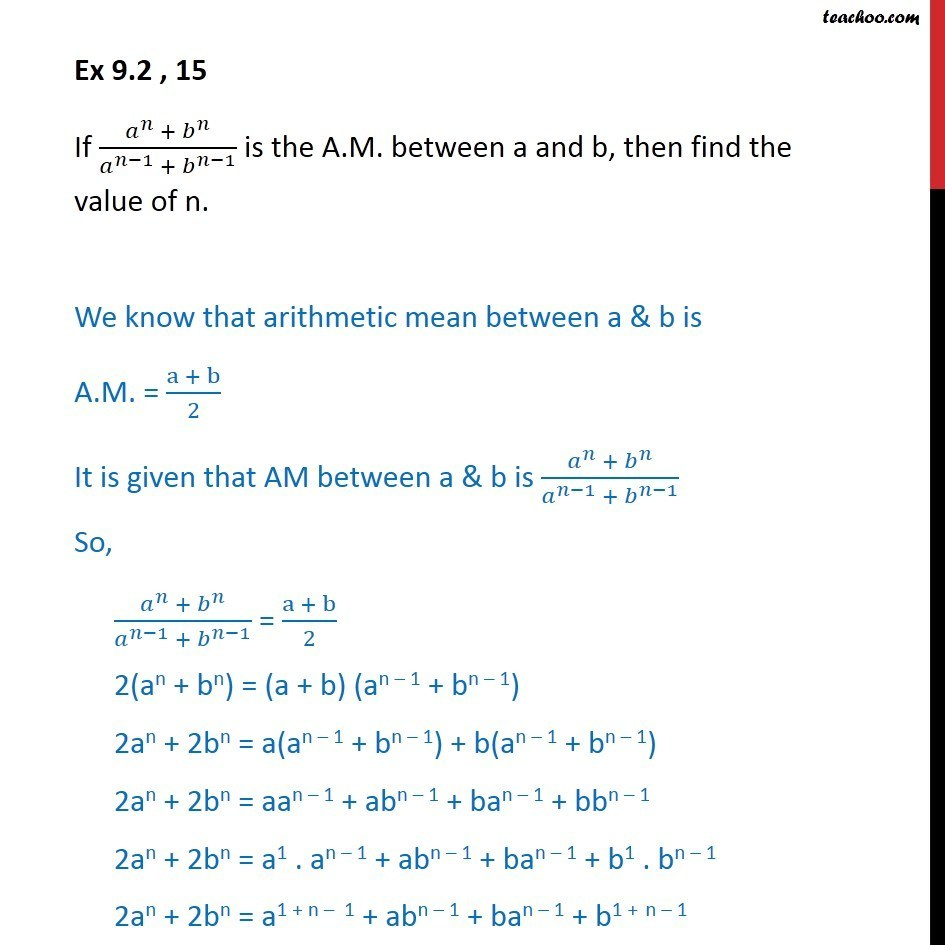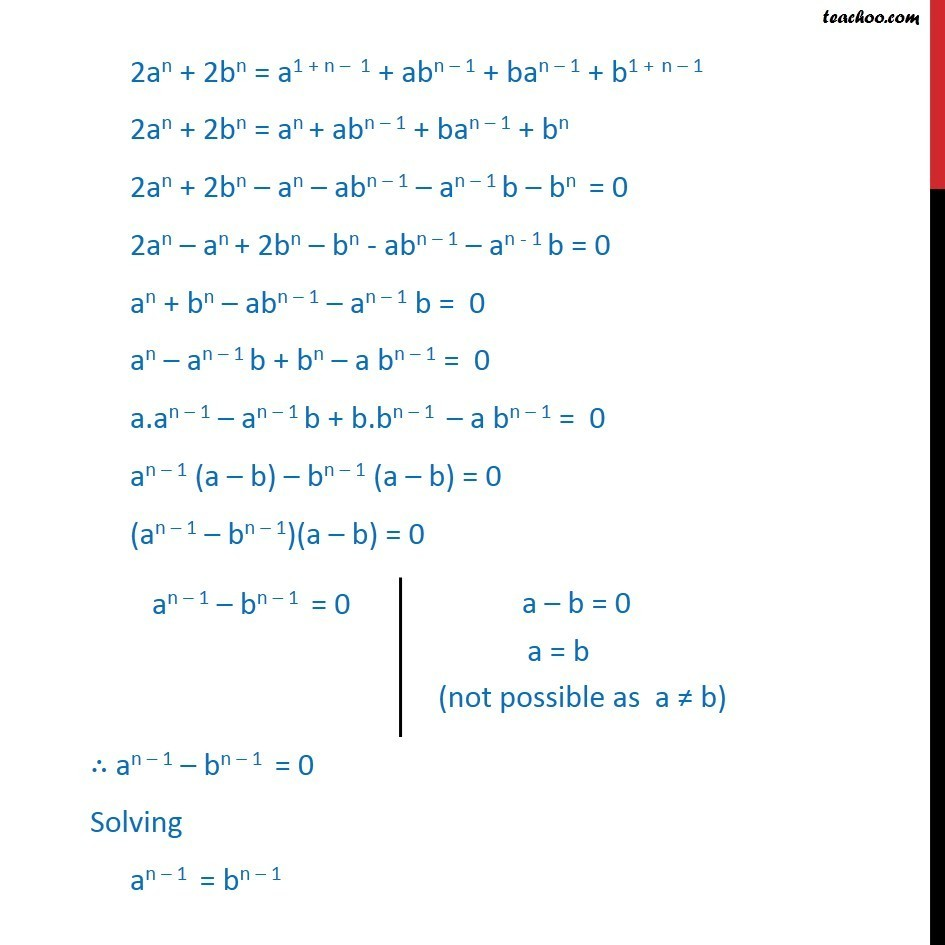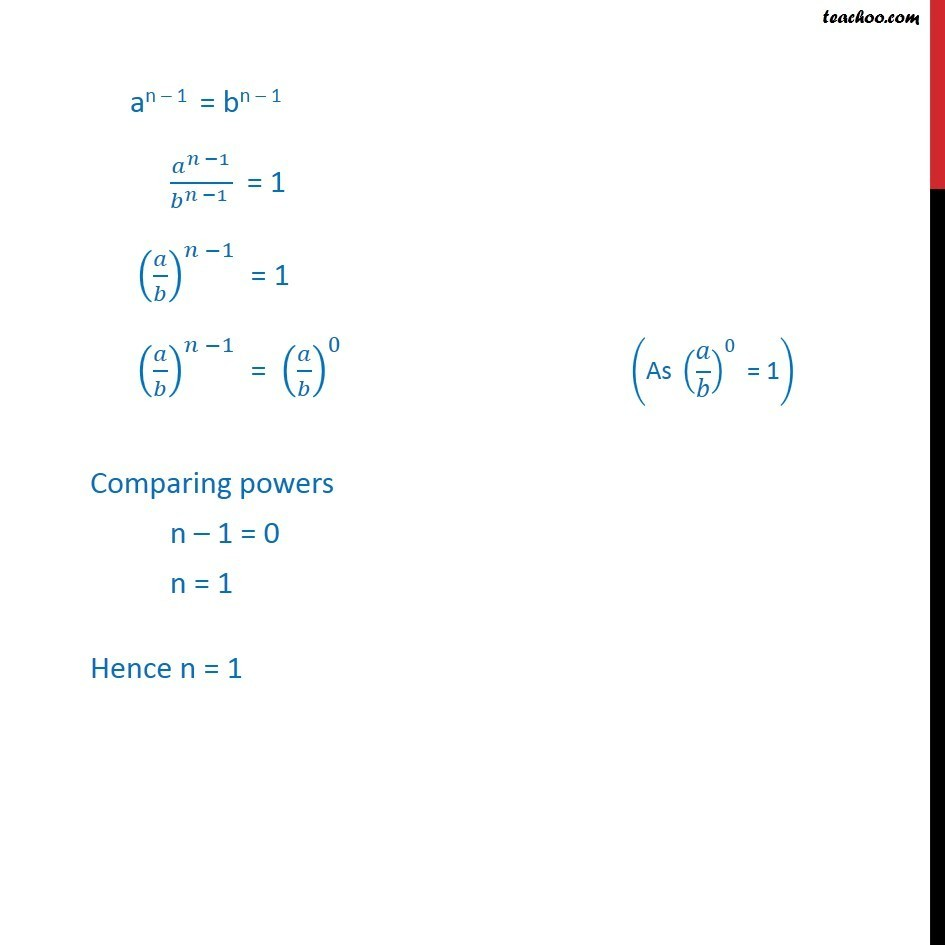Chapter 8 Class 11 Sequences and Series
Concept wiseLearn in your speed, with individual attention - Teachoo Maths 1-on-1 Class

### Transcript

Ex 9.2 , 15 If (𝑎^𝑛 + 𝑏^𝑛)/(𝑎^(𝑛−1) + 𝑏^(𝑛−1) ) is the A.M. between a and b, then find the value of n. We know that arithmetic mean between a & b is A.M. = (a + b)/2 It is given that AM between a & b is (𝑎^𝑛 + 𝑏^𝑛)/(𝑎^(𝑛−1) + 𝑏^(𝑛−1) ) So, (𝑎^𝑛 + 𝑏^𝑛)/(𝑎^(𝑛−1) + 𝑏^(𝑛−1) ) = (a + b)/2 2(an + bn) = (a + b) (an – 1 + bn – 1) 2an + 2bn = a(an – 1 + bn – 1) + b(an – 1 + bn – 1) 2an + 2bn = aan – 1 + abn – 1 + ban – 1 + bbn – 1 2an + 2bn = a1 . an – 1 + abn – 1 + ban – 1 + b1 . bn – 1 2an + 2bn = a1 + n – 1 + abn – 1 + ban – 1 + b1 + n – 1 2an + 2bn = a1 + n – 1 + abn – 1 + ban – 1 + b1 + n – 1 2an + 2bn = an + abn – 1 + ban – 1 + bn 2an + 2bn – an – abn – 1 – an – 1 b – bn = 0 2an – an + 2bn – bn - abn – 1 – an - 1 b = 0 an + bn – abn – 1 – an – 1 b = 0 an – an – 1 b + bn – a bn – 1 = 0 a.an – 1 – an – 1 b + b.bn – 1 – a bn – 1 = 0 an – 1 (a – b) – bn – 1 (a – b) = 0 (an – 1 – bn – 1)(a – b) = 0 ∴ an – 1 – bn – 1 = 0 Solving an – 1 = bn – 1 an – 1 = bn – 1 𝑎^(𝑛 −1)/(𝑏^(𝑛 −1) ) = 1 (𝑎/𝑏)^(𝑛 −1) = 1 (𝑎/𝑏)^(𝑛 −1) = (𝑎/𝑏)^0 Comparing powers n – 1 = 0 n = 1 Hence n = 1